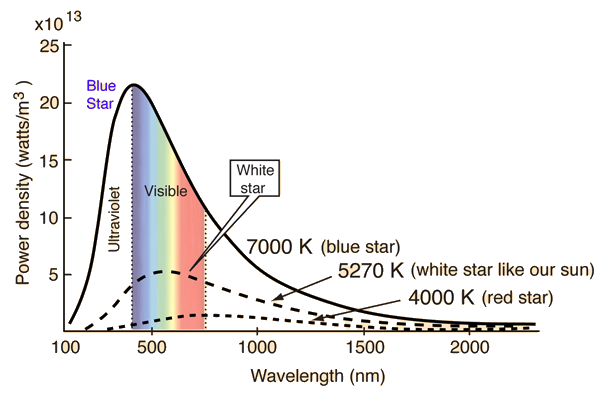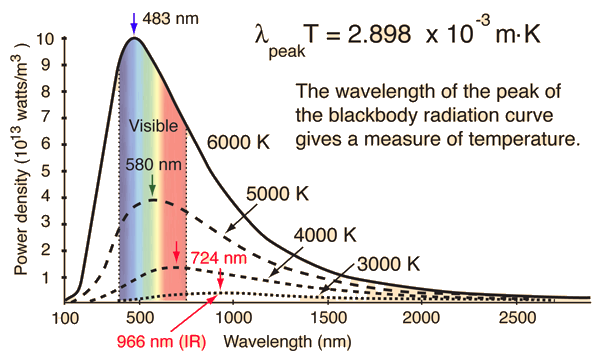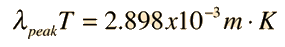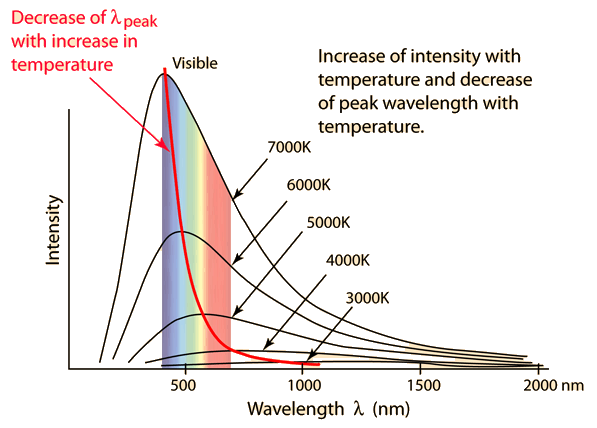# Star Temperatures

Stars approximate blackbody radiators and their visible color depends upon the temperature of the radiator. The curves show blue, white, and red stars. The white star is adjusted to 5270K so that the peak of its blackbody curve is at the peak wavelength of the sun, 550 nm. From the wavelength at the peak, the temperature can be deduced from the Wien displacement law.The change in the visual color of stars of different temperatures can be traced out on a chromaticity diagram.

### Star classification

Index

 HyperPhysics***** Quantum Physics R Nave
Go Back

# Wien's Displacement Law

When the temperature of a blackbody radiator increases, the overall radiated energy increases and the peak of the radiation curve moves to shorter wavelengths. When the maximum is evaluated from the Planck radiation formula, the product of the peak wavelength and the temperature is found to be a constant.Calculation

This relationship is called Wien's displacement law and is useful for determining the temperatures of hot radiant objects such as stars, and indeed for a determination of the temperature of any radiant object whose temperature is far above that of its surroundings.

It should be noted that the peak of the radiation curve in the Wien relationship is the peak only because the intensity is plotted as a function of wavelength. If frequency or some other variable is used on the horizontal axis, the peak will be at a different wavelength.

 Peak of intensity vs frequency plot

### Intensity as a function of temperature and wavelength

Index

 HyperPhysics***** Quantum Physics R Nave
Go Back

# Wien's Displacement Law

For a blackbody radiator, the temperature can be found from the wavelength at which the radiation curve peaks.Discussion

If the temperature is = C = K, then the wavelength at which the radiation curve peaks is:

λpeak = x10^m = nm = microns.

This wavelength corresponds to quantum energy
hν = x 10^ eV.

 Region ofspectrum Wavelength λ Photon Energy RF >1m <10-5 eV microwave, mm 1-0.0003m 10-5-10-2 eV IR 0.0003m-700nm 10-2-1.7eV visible 700-400nm 1.77-3.1eV UV 400-10nm 3.1-120 eV X-ray <10nm >120 eV

 Electromagnetic Spectrum Photon Quantum Energy
Index

 HyperPhysics***** Quantum Physics R Nave
Go BackThe wavelength of the peak of the blackbody radiation curve decreases in a linear fashion as the temperature is increased (Wien's displacement law). This linear variation is not evident in this kind of plot since the intensity increases with the fourth power of the temperature (Stefan- Boltzmann law). The nature of the peak wavelength change is made more evident by plotting the fourth root of the intensity.
Index

 HyperPhysics***** Quantum Physics R Nave
Go Back